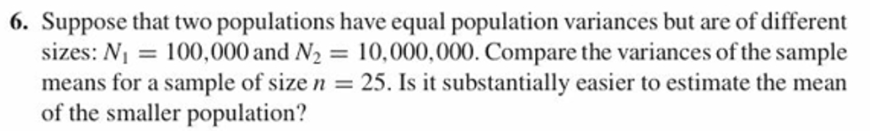6. Suppose that two populations have equal population variances but are of different10,000,000. Compare the variances of the sample25. Is it substantially easier to estimate the meansizes: N100,000 and N2means for a sample of sizeof the smaller population?

Questionhelp_outlineImage Transcriptionclose6. Suppose that two populations have equal population variances but are of different 10,000,000. Compare the variances of the sample 25. Is it substantially easier to estimate the mean sizes: N100,000 and N2 means for a sample of size of the smaller population? fullscreen
Step 1

Given that two populations have equal population variances but different have different sizes N1 = 100000 N2 = 10000000.  A sample of size n= 25 is selected.

The relationship between population variance (σ2) and sample variance (S2 ) is shown below. n is the sample size.

Step 2

Since here the sample size is same in both samples drawn from two populations and bot...

Want to see the full answer?

See Solution

Want to see this answer and more?

Our solutions are written by experts, many with advanced degrees, and available 24/7

See Solution
Tagged in

Measures